$$\newcommand{\id}{\mathrm{id}}$$ $$\newcommand{\Span}{\mathrm{span}}$$ $$\newcommand{\kernel}{\mathrm{null}\,}$$ $$\newcommand{\range}{\mathrm{range}\,}$$ $$\newcommand{\RealPart}{\mathrm{Re}}$$ $$\newcommand{\ImaginaryPart}{\mathrm{Im}}$$ $$\newcommand{\Argument}{\mathrm{Arg}}$$ $$\newcommand{\norm}{\| #1 \|}$$ $$\newcommand{\inner}{\langle #1, #2 \rangle}$$ $$\newcommand{\Span}{\mathrm{span}}$$

# 6.2E: Exercises for Section 6.2

•• OpenStax
• OpenStax
$$\newcommand{\vecs}{\overset { \rightharpoonup} {\mathbf{#1}} }$$ $$\newcommand{\vecd}{\overset{-\!-\!\rightharpoonup}{\vphantom{a}\smash {#1}}}$$$$\newcommand{\id}{\mathrm{id}}$$ $$\newcommand{\Span}{\mathrm{span}}$$ $$\newcommand{\kernel}{\mathrm{null}\,}$$ $$\newcommand{\range}{\mathrm{range}\,}$$ $$\newcommand{\RealPart}{\mathrm{Re}}$$ $$\newcommand{\ImaginaryPart}{\mathrm{Im}}$$ $$\newcommand{\Argument}{\mathrm{Arg}}$$ $$\newcommand{\norm}{\| #1 \|}$$ $$\newcommand{\inner}{\langle #1, #2 \rangle}$$ $$\newcommand{\Span}{\mathrm{span}}$$ $$\newcommand{\id}{\mathrm{id}}$$ $$\newcommand{\Span}{\mathrm{span}}$$ $$\newcommand{\kernel}{\mathrm{null}\,}$$ $$\newcommand{\range}{\mathrm{range}\,}$$ $$\newcommand{\RealPart}{\mathrm{Re}}$$ $$\newcommand{\ImaginaryPart}{\mathrm{Im}}$$ $$\newcommand{\Argument}{\mathrm{Arg}}$$ $$\newcommand{\norm}{\| #1 \|}$$ $$\newcommand{\inner}{\langle #1, #2 \rangle}$$ $$\newcommand{\Span}{\mathrm{span}}$$

1) Derive the formula for the volume of a sphere using the slicing method.

2) Use the slicing method to derive the formula for the volume of a cone.

3) Use the slicing method to derive the formula for the volume of a tetrahedron with side length $$a.$$

4) Use the disk method to derive the formula for the volume of a trapezoidal cylinder.

5) Explain when you would use the disk method versus the washer method. When are they interchangeable?

## Volumes by Slicing

For exercises 6 - 10, draw a typical slice and find the volume using the slicing method for the given volume.

6) A pyramid with height 6 units and square base of side 2 units, as pictured here.Solution:
Here the cross-sections are squares taken perpendicular to the $$y$$-axis.
We use the vertical cross-section of the pyramid through its center to obtain an equation relating $$x$$ and $$y$$.
Here this would be the equation, $$y = 6 - 6x$$. Since we need the dimensions of the square at each $$y$$-level, we solve this equation for $$x$$ to get, $$x = 1 - \tfrac{y}{6}$$.
This is half the distance across the square cross-section at the $$y$$-level, so the side length of the square cross-section is, $$s = 2\left(1 - \tfrac{y}{6}\right).$$
Thus, we have the area of a cross-section is,

$$A(y) = \left[2\left(1 - \tfrac{y}{6}\right)\right]^2 = 4\left(1 - \tfrac{y}{6}\right)^2.$$

\begin{align*} \text{Then},\quad V &= \int_0^6 4\left(1 - \tfrac{y}{6}\right)^2 \, dy \\[5pt] &= -24 \int_1^0 u^2 \, du, \quad \text{where} \, u = 1 - \tfrac{y}{6}, \, \text{so} \, du = -\tfrac{1}{6}\,dy, \quad \implies \quad -6\,du = dy \\[5pt] &= 24 \int_0^1 u^2 \, du = 24\dfrac{u^3}{3}\bigg|_0^1 \\[5pt] &= 8u^3\bigg|_0^1 \\[5pt] &= 8\left( 1^3 - 0^3 \right) \quad= \quad 8\, \text{units}^3 \end{align*}

7) A pyramid with height 4 units and a rectangular base with length 2 units and width 3 units, as pictured here.8) A tetrahedron with a base side of 4 units,as seen here.$$V = \frac{32}{3\sqrt{2}} = \frac{16\sqrt{2}}{3}$$ units3

9) A pyramid with height 5 units, and an isosceles triangular base with lengths of 6 units and 8 units, as seen here.10) A cone of radius $$r$$ and height $$h$$ has a smaller cone of radius $$r/2$$ and height $$h/2$$ removed from the top, as seen here. The resulting solid is called a frustum.$$V = \frac{7\pi}{12} hr^2$$ units3

For exercises 11 - 16, draw an outline of the solid and find the volume using the slicing method.

11) The base is a circle of radius $$a$$. The slices perpendicular to the base are squares.

12) The base is a triangle with vertices $$(0,0),(1,0),$$ and $$(0,1)$$. Slices perpendicular to the $$xy$$-plane are semicircles.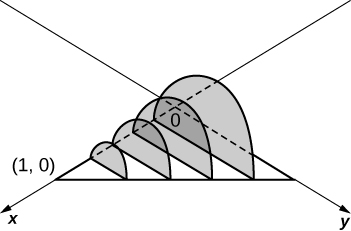$$\displaystyle V = \int_0^1 \frac{\pi(1-x)^2}{8}\, dx \quad = \quad \frac{π}{24}$$ units3

13) The base is the region under the parabola $$y=1−x^2$$ in the first quadrant. Slices perpendicular to the $$xy$$-plane are squares.

14) The base is the region under the parabola $$y=1−x^2$$ and above the $$x$$-axis. Slices perpendicular to the $$y$$-axis are squares.$$\displaystyle V = \int_0^1 4(1 - y)\,dy \quad = \quad 2$$ units3

15) The base is the region enclosed by $$y=x^2)$$ and $$y=9.$$ Slices perpendicular to the $$x$$-axis are right isosceles triangles.

16) The base is the area between $$y=x$$ and $$y=x^2$$. Slices perpendicular to the $$x$$-axis are semicircles.$$\displaystyle V = \int_0^1 \frac{\pi}{8}\left( x - x^2 \right)^2 \, dx \quad=\quad \frac{π}{240}$$ units3

## Disk and Washer Method

For exercises 17 - 24, draw the region bounded by the curves. Then, use the disk or washer method to find the volume when the region is rotated around the $$x$$-axis.

17) $$x+y=8,\quad x=0$$, and $$y=0$$

18) $$y=2x^2,\quad x=0,\quad x=4,$$ and $$y=0$$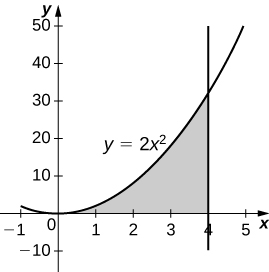$$\displaystyle V = \int_0^4 4\pi x^4\, dx \quad=\quad \frac{4096π}{5}$$ units3

19) $$y=e^x+1,\quad x=0,\quad x=1,$$ and $$y=0$$

20) $$y=x^4,\quad x=0$$, and $$y=1$$$$\displaystyle V = \int_0^1 \pi\left( 1^2 - \left( x^4\right)^2\right)\, dx = \int_0^1 \pi\left( 1 - x^8\right)\, dx \quad = \quad \frac{8π}{9}$$ units3

21) $$y=\sqrt{x},\quad x=0,\quad x=4,$$ and $$y=0$$

22) $$y=\sin x,\quad y=\cos x,$$ and $$x=0$$$$\displaystyle V = \int_0^{\pi/4} \pi \left( \cos^2 x - \sin^2 x\right) \, dx = \int_0^{\pi/4} \pi \cos 2x \, dx \quad=\quad \frac{π}{2}$$ units3

23) $$y=\dfrac{1}{x},\quad x=2$$, and $$y=3$$

24) $$x^2−y^2=9$$ and $$x+y=9,\quad y=0$$ and $$x=0$$$$V = 207π$$ units3

For exercises 25 - 32, draw the region bounded by the curves. Then, find the volume when the region is rotated around the $$y$$-axis.

25) $$y=4−\dfrac{1}{2}x,\quad x=0,$$ and $$y=0$$

26) $$y=2x^3,\quad x=0,\quad x=1,$$ and $$y=0$$$$V = \frac{4π}{5}$$ units3

27) $$y=3x^2,\quad x=0,$$ and $$y=3$$

28) $$y=\sqrt{4−x^2},\quad y=0,$$ and $$x=0$$$$V = \frac{16π}{3}$$ units3

29) $$y=\dfrac{1}{\sqrt{x+1}},\quad x=0$$, and $$x=3$$

30) $$x=\sec(y)$$ and $$y=\dfrac{π}{4},\quad y=0$$ and $$x=0$$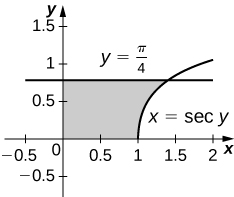$$V = π$$ units3

31) $$y=\dfrac{1}{x+1},\quad x=0$$, and $$x=2$$

32) $$y=4−x,\quad y=x,$$ and $$x=0$$$$V = \frac{16π}{3}$$ units3

For exercises 33 - 40, draw the region bounded by the curves. Then, find the volume when the region is rotated around the $$x$$-axis.

33) $$y=x+2,\quad y=x+6,\quad x=0$$, and $$x=5$$

34) $$y=x^2$$ and $$y=x+2$$$$V = \frac{72π}{5}$$ units3

35) $$x^2=y^3$$ and $$x^3=y^2$$

36) $$y=4−x^2$$ and $$y=2−x$$$$V = \frac{108π}{5}$$ units3

37) [T] $$y=\cos x,\quad y=e^{−x},\quad x=0$$, and $$x=1.2927$$

38) $$y=\sqrt{x}$$ and $$y=x^2$$$$V = \frac{3π}{10}$$ units3

39) $$y=\sin x,\quad y=5\sin x,\quad x=0$$ and $$x=π$$

40) $$y=\sqrt{1+x^2}$$ and $$y=\sqrt{4−x^2}$$$$V = 2\sqrt{6}π$$ units3

For exercises 41 - 45, draw the region bounded by the curves. Then, use the washer method to find the volume when the region is revolved around the $$y$$-axis.

41) $$y=\sqrt{x},\quad x=4$$, and $$y=0$$

42) $$y=x+2,\quad y=2x−1$$, and $$x=0$$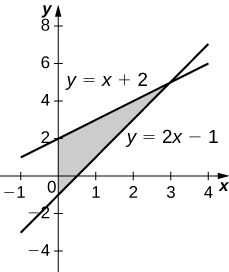$$V = 9π$$ units3

43) $$y=\dfrac{3}{x}$$ and $$y=x^3$$

44) $$x=e^{2y},\quad x=y^2,\quad y=0$$, and $$y=\ln(2)$$$$V = \dfrac{π}{20}(75−4\ln^5(2))$$ units3

45) $$x=\sqrt{9−y^2},\quad x=e^{−y},\quad y=0$$, and $$y=3$$

46) Yogurt containers can be shaped like frustums. Rotate the line $$y=\left(\frac{1}{m}\right)x$$ around the $$y$$-axis to find the volume between $$y=a$$ and $$y=b$$.$$V = \dfrac{m^2π}{3}(b^3−a^3)$$ units3

47) Rotate the ellipse $$\dfrac{x^2}{a^2}+\dfrac{y^2}{b^2}=1$$ around the $$x$$-axis to approximate the volume of a football, as seen here.48) Rotate the ellipse $$\dfrac{x^2}{a^2}+\dfrac{y^2}{b^2}=1$$ around the $$y$$-axis to approximate the volume of a football.

$$V = \frac{4a^2bπ}{3}$$ units3

49) A better approximation of the volume of a football is given by the solid that comes from rotating $$y=\sin x) around the \(x$$-axis from $$x=0$$ to $$x=π$$. What is the volume of this football approximation, as seen here?For exercises 51 - 56, find the volume of the solid described.

51) The base is the region between $$y=x$$ and $$y=x^2$$. Slices perpendicular to the $$x$$-axis are semicircles.

52) The base is the region enclosed by the generic ellipse $$\dfrac{x^2}{a^2}+\dfrac{y^2}{b^2}=1.$$ Slices perpendicular to the $$x$$-axis are semicircles.

$$V = \frac{2ab^2π}{3}$$ units3

53) Bore a hole of radius a down the axis of $$a$$ right cone and through the base of radius $$b$$, as seen here.54) Find the volume common to two spheres of radius $$r$$ with centers that are $$2h$$ apart, as shown here.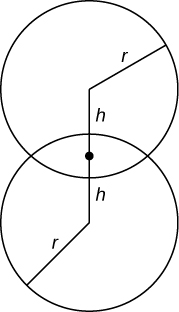$$V = \frac{π}{12}(r+h)^2(6r−h)$$ units3

55) Find the volume of a spherical cap of height $$h$$ and radius $$r$$ where $$h<r$$, as seen here.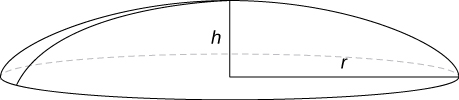56) Find the volume of a sphere of radius $$R$$ with a cap of height $$h$$ removed from the top, as seen here.$$V = \dfrac{π}{3}(h+R)(h−2R)^2$$ units3